Poll has expired, here are the final results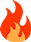554°
• 1371
• 52
•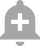• Last Comment
Garyhost

Q.1) 9-4+1
A) 6
B) 4

Q2) 6÷2(2+1)
A) 1
B) 9

Just for fun...♥️

2. B) 9

20 Dimers
• Sort By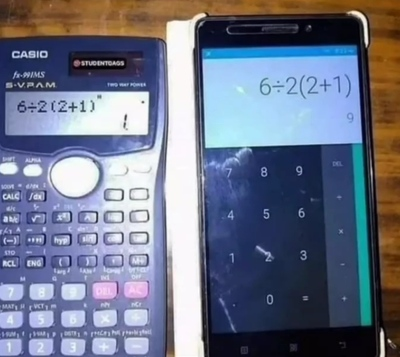*Don't use calculator

1. 4

2. 9

BODMAS

4 , 1

1.4

2.1

A/c "BODMAS"

1

4,9

Update - 6,9

kartikxxx wrote:

4,9

1. 9-4+1

9-5= 4

2. 6÷2(2+1)

6÷4+2

6÷6 = 1

Update : 6,9

I realised this after poll submission

So, 6÷2(2+1)

First we solve bracket :

= 6÷2(3) = 6÷2×3

Divide first then multiply :

= 3×3 = 9

tappukepapa wrote:

1. 9-4+1

9-5= 4

2. 6÷2(2+1)

6÷4+2

6÷6 = 1

Q1. 9-4+1

Rearrange:

=9+1-4

=6

Deal_of_the_day wrote:

It's 4,9 not 4,1

I realised this after poll submission

So, 6÷2(2+1)

First we solve bracket :

= 6÷2(3) = 6÷2×3

Divide first then multiply :

= 3×3 = 9

*Dumb

Expand
G0KU wrote:

*Dumb

Who?

1.A) 6
Edit: 2.A) 9 is correct

Expand
quantum wrote:

Q1. 9-4+1

Rearrange:

=9+1-4

=6

Understood, thanks !

violin wrote:

1.A) 6

2.A) 1

Not 1,

9 is the correct as "Bracket rule" of BODMAS applies  INSIDE the bracket and not OUTSIDE the bracket.

After Addition, singularity existed in numerical terms, the bracket itself converted into multiplication.

Expand
quantum wrote:

Q1. 9-4+1

Rearrange:

=9+1-4

=6

Explain pls.

Expand
quantum wrote:

Not 1,

9 is the correct as "Bracket rule" of BODMAS applies  INSIDE the bracket and not OUTSIDE the bracket.

After Addition, singularity existed in numerical terms, the bracket itself converted into multiplication.

yes, 9 is correct
Expand
tappukepapa wrote:

Explain pls.

By BODM AS [rule]
Q1. 9-4+1

so, positive integers are 9 and 1
and, negative integer is 4

So, by rule

now, use detrimental integer "4" after all addition is over.
So, 10-4=6

or use rearranging method,
which used, where a similar number(+/-) are placed together
Expand
quantum wrote:
By BODM AS [rule]
Q1. 9-4+1

so, positive integers are 9 and 1
and, negative integer is 4

So, by rule

now, use detrimental integer "4" after all addition is over.
So, 10-4=6

or use rearranging method,
which used, where a similar number(+/-) are placed together

OR simply solve from left to right because addition and subtraction have same level of precedence.

9-4+1 = 5+1 = 6

Expand
Deal_of_the_day wrote:

OR simply solve from left to right because addition and subtraction have same level of precedence.

9-4+1 = 5+1 = 6

You can't. It'll lead to disproportionate equations in future. Not the right approach.
Expand
quantum wrote:
You can't. It'll lead to disproportionate equations in future. Not the right approach.

Can you show some examples?

Expand
Deal_of_the_day wrote:

Can you show some examples?

No, but functions are always rule based. So, if not done according to the rule, anomalies can be created. (Just an op-in-ion)
If there is any postulate, then I have no issues as Euclid and Dalton messed with them in mah lyf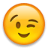🤣
Expand
quantum wrote:
By BODM AS [rule]
Q1. 9-4+1

so, positive integers are 9 and 1
and, negative integer is 4

So, by rule

now, use detrimental integer "4" after all addition is over.
So, 10-4=6

or use rearranging method,
which used, where a similar number(+/-) are placed together

Is this actually accepted everywhere?

They are teaching wrong in schools unfortunately, as far as I remember. Because they taught BODMAS before integers.

Never took interest in maths, but this problem is intriguing.

Logically the answer is correct, but how can the system be so wrong and then expect students to be geniuses.

Expand
androgame wrote:

Is this actually accepted everywhere?

They are teaching wrong in schools unfortunately, as far as I remember. Because they taught BODMAS before integers.

Never took interest in maths, but this problem is intriguing.

Logically the answer is correct, but how can the system be so wrong and then expect students to be geniuses.

mhare to mas saab(teacher) aate the chhari leke, so yaad ni kya padhya pehle. sarkari school was lit.

btw number line (not named integer, but yeah similar) came first then add/subs/mult/div. (i think) 🧐

@androgame you lied, who saw 14 million possibilities 🤣🤣 to be precise 14,000,605

Expand
quantum wrote:
By BODM AS [rule]
Q1. 9-4+1

so, positive integers are 9 and 1
and, negative integer is 4

So, by rule

now, use detrimental integer "4" after all addition is over.
So, 10-4=6

or use rearranging method,
which used, where a similar number(+/-) are placed together

9-4+1

Ramu ki daadi ne Ramu ko 9 rupay diye 😀

Shamu ne dekh liya, bola chal lauta mere 4 rupay ☹️

Shamu ki nikkar ki jeb fati thi toh usmein se ek ka sikka gir gaya 🤫

Ramu ne utha liya 😀

Kahaani khatm, paisa hazam

Expand
saucap wrote:

9-4+1

Ramu ki daadi ne Ramu ko 9 rupay diye 😀

Shamu ne dekh liya, bola chal lauta mere 4 rupay ☹️

Shamu ki nikkar ki jeb fati thi toh usmein se ek ka sikka gir gaya 🤫

Ramu ne utha liya 😀

Kahaani khatm, paisa hazam

Shamu ki nikkar ki jeb fati thi toh usmein se ek ka sikka gir gaya
Ye suit ni kiya,
+9
-4
+1 (Ramu ne ek rupaya gira dekha [jo iss kahani mein kirdaar na ho uska])
Shamu se lega ya Shamu ka lega *rupaya to appropriate nahi hoga 🧐
Expand
quantum wrote:
Shamu ki nikkar ki jeb fati thi toh usmein se ek ka sikka gir gaya
Ye suit ni kiya,
+9
-4
+1 (Ramu ne ek rupaya gira dekha [jo iss kahani mein kirdaar na ho uska])
Shamu se lega ya Shamu ka lega *rupaya to appropriate nahi hoga 🧐

Arey! Ramu hero hai, Shamu villain hai. End mein Hero hi jeetata hai

Expand
androgame wrote:

Is this actually accepted everywhere?

They are teaching wrong in schools unfortunately, as far as I remember. Because they taught BODMAS before integers.

Never took interest in maths, but this problem is intriguing.

Logically the answer is correct, but how can the system be so wrong and then expect students to be geniuses.

I knew my maths syllabus and esp our maths teacher was 💩

But my mom never believed me 🥲

Garyhost wrote: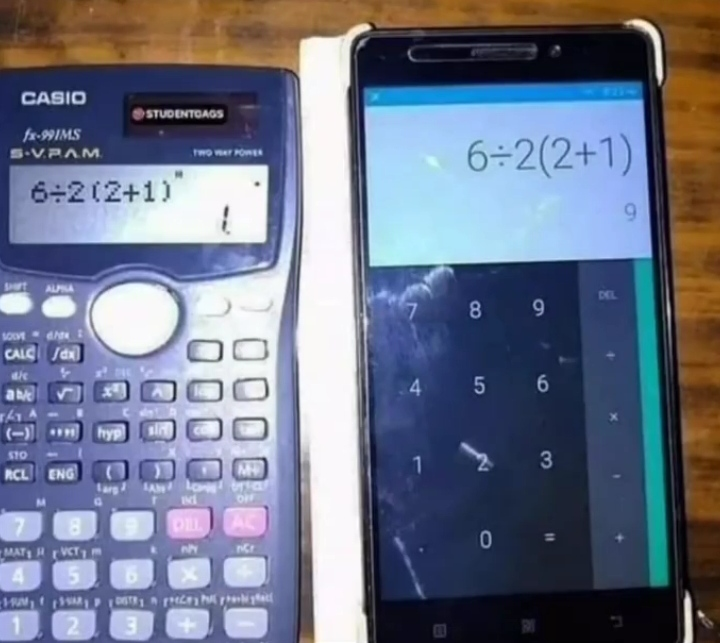*Don't use calculator

😀😀.Second answer should be 9.Throw the calculator on left side to dustbin 🗑️🗑️.It's fake calculator.According to BODMAS ,second answer should be 9.Hottest Discussions
1116° (Upcoming) Apple iPhone 13 Under 39k in Amazon Great Indian Festival 2023dealskabap
59
1651° All Bank Credit Card Offers Official Link Compilationshraaj
33
1401° Flipkart VIP Membership @ 499sumukha679776
75
133° HDFC Bank sent SMS for linking of RuPay CC - Potential Fraud/Scam or Glitch?sahil1033
14
212° Carpooling is illegal; Rs 10,000 challan implementedBk100
18
View all